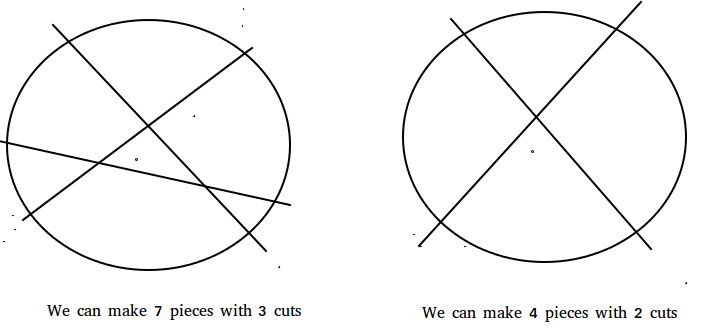Related Articles

# The Lazy Caterer’s Problem

• Difficulty Level : Easy
• Last Updated : 30 Mar, 2021

Given an integer n, denoting the number of cuts that can be made on a pancake, find the maximum number of pieces that can be formed by making n cuts.
Examples :

```Input :  n = 1
Output : 2
With 1 cut we can divide the pancake in 2 pieces

Input :  2
Output : 4
With 2 cuts we can divide the pancake in 4 pieces

Input : 3
Output : 7
We can divide the pancake in 7 parts with 3 cuts

Input : 50
Output : 1276
```Attention reader! Don’t stop learning now. Get hold of all the important mathematical concepts for competitive programming with the Essential Maths for CP Course at a student-friendly price. To complete your preparation from learning a language to DS Algo and many more,  please refer Complete Interview Preparation Course.

```Let f(n) denote the maximum number of pieces
that can be obtained by making n cuts.
Trivially,
f(0) = 1
As there'd be only 1 piece without any cut.

Similarly,
f(1) = 2

Proceeding in similar fashion we can deduce
the recursive nature of the function.
The function can be represented recursively as :
f(n) = n + f(n-1)

Hence a simple solution based on the above
formula can run in O(n). ```

We can optimize above formula.

```We now know ,
f(n) = n + f(n-1)

Expanding f(n-1) and so on we have ,
f(n) = n + n-1 + n-2 + ...... + 1 + f(0)

which gives,
f(n) = (n*(n+1))/2 + 1```

Hence with this optimization, we can answer all the queries in O(1).
Below is the implementation of above idea :

## C++

 `// A C++ program to find the solution to``// The Lazy Caterer's Problem``#include ``using` `namespace` `std;` `// This function receives an integer n``// and returns the maximum number of``// pieces that can be made form pancake``// using n cuts``int` `findPieces(``int` `n)``{``    ``// Use the formula``    ``return` `(n * ( n + 1)) / 2 + 1;``}` `// Driver Code``int` `main()``{``    ``cout << findPieces(1) << endl;``    ``cout << findPieces(2) << endl;``    ``cout << findPieces(3) << endl;``    ``cout << findPieces(50) << endl;``    ``return` `0;``}`

## Java

 `// Java program to find the solution to``// The Lazy Caterer's Problem``import` `java.io.*;` `class` `GFG``{``    ``// This function returns the maximum``    ``// number of pieces that can be made``    ``//  form pancake using n cuts``    ``static` `int` `findPieces(``int` `n)``    ``{``        ``// Use the formula``        ``return` `(n * (n + ``1``)) / ``2` `+ ``1``;``    ``}``    ` `    ``// Driver program to test above function``    ``public` `static` `void` `main (String[] args)``    ``{``        ``System.out.println(findPieces(``1``));``        ``System.out.println(findPieces(``2``));``        ``System.out.println(findPieces(``3``));``        ``System.out.println(findPieces(``50``));``    ``}``}` `// This code is contributed by Pramod Kumar`

## Python3

 `# A Python 3 program to``# find the solution to``# The Lazy Caterer's Problem` `# This function receives an``# integer n and returns the``# maximum number of pieces``# that can be made form``# pancake using n cuts``def` `findPieces( n ):` `    ``# Use the formula``    ``return` `(n ``*` `( n ``+` `1``)) ``/``/` `2` `+` `1` `# Driver Code``print``(findPieces(``1``))``print``(findPieces(``2``))``print``(findPieces(``3``))``print``(findPieces(``50``))` `# This code is contributed``# by ihritik`

## C#

 `// C# program to find the solution``// to The Lazy Caterer's Problem``using` `System;` `class` `GFG``{``    ``// This function returns the maximum``    ``// number of pieces that can be made``    ``// form pancake using n cuts``    ``static` `int` `findPieces(``int` `n)``    ``{``        ``// Use the formula``        ``return` `(n * (n + 1)) / 2 + 1;``    ``}``    ` `    ``// Driver code``    ``public` `static` `void` `Main ()``    ``{``        ``Console.WriteLine(findPieces(1));``        ``Console.WriteLine(findPieces(2));``        ``Console.WriteLine(findPieces(3));``        ``Console.Write(findPieces(50));``    ``}``}` `// This code is contributed by Nitin Mittal.`

## PHP

 ``

## Javascript

 ``

Output :

```2
4
7
1276```

References : oeis.org
This article is contributed by Ashutosh Kumar .If you like GeeksforGeeks and would like to contribute, you can also write an article using contribute.geeksforgeeks.org or mail your article to contribute@geeksforgeeks.org. See your article appearing on the GeeksforGeeks main page and help other Geeks.